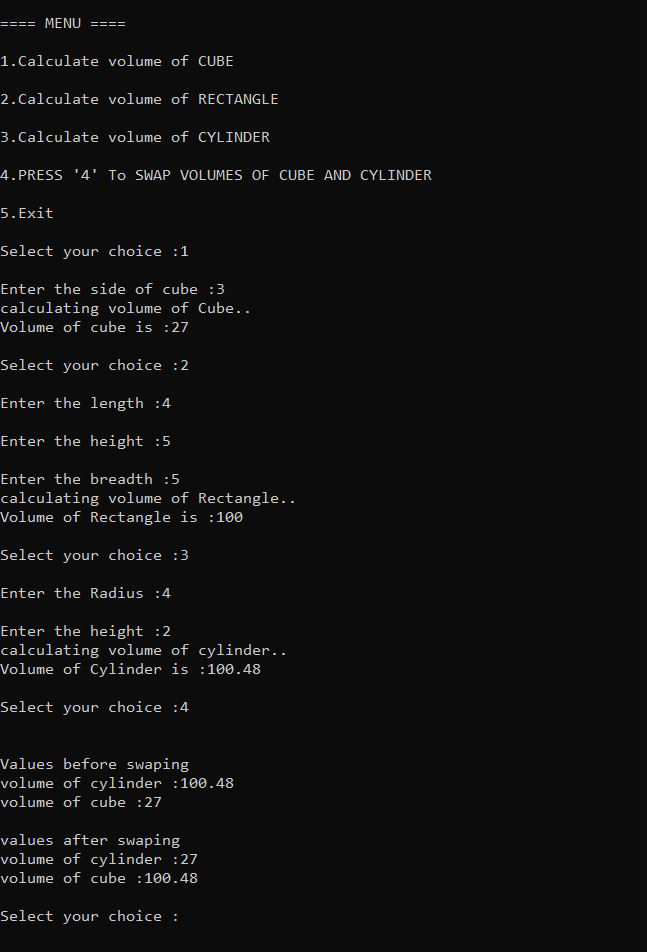# Menu-Based program to find the volume of 3D shapes using function overloading

• Difficulty Level : Basic
• Last Updated : 25 May, 2022

Given the dimensions of the 3D Shapes like Cube, Cuboid, or Cylinder, the task is to find the volume of all the 3D Shapes using function overloading.

Examples:

Input: Cube: L = 3, Cuboid: L = 3, B = 4, H = 3, Cylinder: R = 2, H = 7
Output:
Volume of Cube: 27
Volume of Cuboid: 36
Volume of Cylinder: 176

Input: Cube: L = 2, Cuboid: L = 3, B = 2, H = 3, Cylinder: R = 1, H = 7
Output:
Volume of Cube: 8
Volume of Cuboid: 18
Volume of Cylinder: 22

Approach: The given problem can be solved by creating the different functions having the same name, say Volume but different function definitions. For each shape and then overload all the functions by passing different parameters to them and then call all the functions from the main function with the help of switch-case statements.

Below is the C++ program to find the volume of the different 3D shapes using function overloading:

## C++

 `// C++ program to find the volume of``// 2D Shapes using the function``// overloading``#include ``#define PI 3.14``using` `namespace` `std;` `// Function to find volume of cube``float` `volume(``float` `side)``{``    ``cout << ``"calculating volume of Cube.."``;``    ``float` `calculate_volume;` `    ``calculate_volume``        ``= side * side * side;` `    ``// Return the volume``    ``return` `calculate_volume;``}` `// Function to find volume of rectangle``float` `volume(``float` `length, ``float` `breadth,``             ``float` `height)``{``    ``cout << ``"calculating volume of "``         ``<< ``"Rectangle.."``;``    ``int` `calculate_volume;``    ``calculate_volume``        ``= length * breadth * height;` `    ``// Return the volume``    ``return` `calculate_volume;``}` `// Function to find volume of cylinder``float` `volume(``double` `radius,``             ``double` `height)``{``    ``cout << ``"calculating volume of"``         ``<< ``" cylinder.."``;``    ``float` `calculate_volume;``    ``calculate_volume``        ``= PI * radius * radius * height;` `    ``// Return the volume``    ``return` `calculate_volume;``}` `// Function to swap the values of``// volume of cube and cylinder``void` `swapvalues(``float` `cylinder, ``float` `cube)``{``    ``float` `third_variable;``    ``if` `(cylinder == 0 || cube == 0) {` `        ``cout << ``"\nvalues are not assign"``;``        ``return``;``    ``}` `    ``cout << ``"\n\nValues before swaping"``;``    ``cout << ``"\nvolume of cylinder :"``         ``<< cylinder;``    ``cout << ``"\nvolume of cube :"``         ``<< cube;` `    ``// Perform Swap Operation``    ``third_variable = cube;``    ``cube = cylinder;``    ``cylinder = third_variable;` `    ``cout << ``"\n\nvalues after swaping "``;` `    ``cout << ``"\nvolume of cylinder :"``         ``<< cylinder;` `    ``cout << ``"\nvolume of cube :"``         ``<< cube;``}` `// Driver Code``int` `main()``{``    ``float` `height, radius, length;``    ``float` `breadth, rectangleHeight;``    ``float` `volumeCube = 0;``    ``float` `volumeRectangle = 0;``    ``float` `volumeCylinder = 0;``    ``float` `side;` `    ``int` `choice;` `    ``// Main menu``    ``cout << ``"\n\n==== MENU ===="``;``    ``cout << ``"\n\n1.Calculate volume "``         ``<< ``"of CUBE"``;``    ``cout << ``"\n\n2.Calculate volume "``         ``<< ``"of RECTANGLE"``;``    ``cout << ``"\n\n3.Calculate volume "``         ``<< ``"of CYLINDER"``;``    ``cout << ``"\n\n4.PRESS '4' To SWAP "``         ``<< ``"VOLUMES OF CUBE AND "``            ``"CYLINDER"``;``    ``cout << ``"\n\n5.Exit"``;` `    ``while` `(1) {` `        ``cout << ``"\n\nSelect your choice :"``;``        ``cin >> choice;` `        ``// Switch Case``        ``switch` `(choice) {` `        ``case` `1:``            ``cout << ``"\nEnter the side"``                 ``<< ``" of cube :"``;``            ``cin >> side;``            ``volumeCube = volume(side);` `            ``cout << ``"\nVolume of cube is :"``                 ``<< volumeCube;``            ``break``;` `        ``case` `2:``            ``cout << ``"\nEnter the length :"``;``            ``cin >> length;` `            ``cout << ``"\nEnter the height :"``;``            ``cin >> rectangleHeight;` `            ``cout << ``"\nEnter the breadth :"``;``            ``cin >> breadth;` `            ``volumeRectangle``                ``= volume(length, breadth,``                         ``rectangleHeight);` `            ``cout << ``"\nVolume of Rectangle is :"``                 ``<< volumeRectangle;` `            ``break``;` `        ``case` `3:``            ``cout << ``"\nEnter the Radius :"``;``            ``cin >> radius;` `            ``cout << ``"\nEnter the height :"``;``            ``cin >> height;` `            ``volumeCylinder = volume(``                ``radius, height);` `            ``cout << ``"\nVolume of Cylinder is :"``                 ``<< volumeCylinder;``            ``break``;` `        ``case` `4:` `            ``swapvalues(volumeCylinder,``                       ``volumeCube);``            ``break``;` `        ``case` `5:``            ``exit``(0);``        ``}``    ``}``      ``return` `0;``}`

Output:My Personal Notes arrow_drop_up